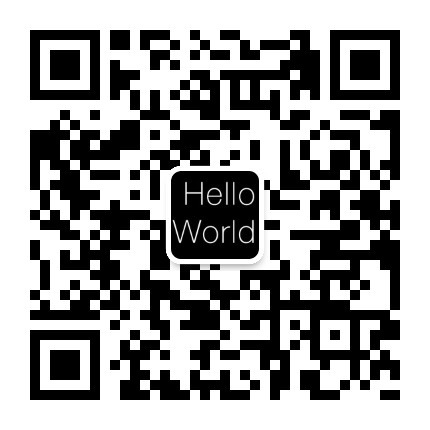/ 读书笔记

# Go语言圣经 学习笔记（六）(第二部分)

## 第六章 方法

### 6.4 方法值和方法表达式

• 我们经常选择一个方法，并且在同一个表达式里执行，比如常见的p.Distance()形式，实际上将其分成两步来执行也是可能的。p.Distance叫作“选择器”，选择器会返回一个方法"值"->一个将方法(Point.Distance)绑定到特定接收器变量的函数
``````p := Point{1, 2}
q := Point{4, 6}
distanceFromP := p.Distance // method value
fmt.Println(distanceFromP(q)) // "5"
``````
• 当T是一个类型时，方法表达式可能会写作T.f或者(*T).f，会返回一个函数"值"，这种函数会将其第一个参数用作接收器，所以可以用通常(译注：不写选择器)的方式来对其进行调用：
``````p := Point{1, 2}
q := Point{4, 6}
distance := Point.Distance // method expression
fmt.Println(distance(p, q)) // "5"
fmt.Printf("%T\n", distance) // "func(Point, Point) float64"
scale := (*Point).ScaleBy
scale(&p, 2)
fmt.Println(p) // "{2 4}"
fmt.Printf("%T\n", scale) // "func(*Point, float64)"
// 译注：这个Distance实际上是指定了Point对象为接收器的一个方法func (p Point) Distance()，
// 但通过Point.Distance得到的函数需要比实际的Distance方法多一个参数，
// 即其需要用第一个额外参数指定接收器，后面排列Distance方法的参数。
// 看起来本书中函数和方法的区别是指有没有接收器，而不像其他语言那样是指有没有返回值。
``````

### 6.5. 示例: Bit数组

• 一个bit数组通常会用一个无符号数或者称之为“字”的slice或者来表示，每一个元素的每一位都表示集合里的一个值。当集合的第i位被设置时，我们才说这个集合包含元素i
``````// An IntSet is a set of small non-negative integers.
// Its zero value represents the empty set.
type IntSet struct {
words []uint64
}
// Has reports whether the set contains the non-negative value x.
func (s *IntSet) Has(x int) bool {
word, bit := x/64, uint(x%64)
return word < len(s.words) && s.words[word]&(1<<bit) != 0
}
func (s *IntSet) Add(x int) {
word, bit := x/64, uint(x%64)
for word >= len(s.words) {
s.words = append(s.words, 0)
}
s.words[word] |= 1 << bit
}
// UnionWith sets s to the union of s and t.
func (s *IntSet) UnionWith(t *IntSet) {
for i, tword := range t.words {
if i < len(s.words) {
s.words[i] |= tword
} else {
s.words = append(s.words, tword)
}
}
}
``````

### 6.6 封装

• 一个对象的变量或者方法如果对调用方是不可见的话，一般就被定义为“封装”
• Go语言只有一种控制可见性的手段：大写首字母的标识符会从定义它们的包中被导出，小写字母的则不会。
• 如果我们想要封装一个对象，我们必须将其定义为一个struct。
• 这种基于名字的手段使得在语言中最小的封装单元是package，而不是像其它语言一样的类型。一个struct类型的字段对同一个包的所有代码都有可见性

#### remote_pluto